# dForms

Concept by Tony Wills

WWW: www.wills-watson.co.uk

Surface modelling using Evolver
by Kenneth A. Brakke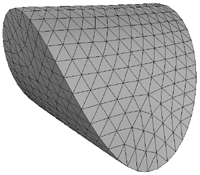Definition

A dForm consists of two 2D shapes cut from a non-elastic material (eg: paper). The two shapes must have identical perimeter length. The 3D dForm is created by choosing a point on each shape for an initial join, the remainder of the perimeters are subsequently joined together. In general, a different 3D shape will result from different initial joining points. Folds may be introduced only if necessary.

dForms are not restricted to convex outlines. However concave outlines often lead to intersecting surfaces and are thus hard to build physically.

Two ellipses

The dForm of two circles is always unexciting no matter where the initial connection is made. The next simplest shape might be considered to be two ellipses. If they are initially joined at the same position on each perimeter then the result is just a flat sheet. However if one ellipse is rotated with respect to the other before the initial join then more interesting solids result. Some computer generated examples are given below for 3 different rotation angles and two different ellipse radius ratios.

 Rotation by 22.5 degrees Ellipse radii are 0.8 and 1.0 [DXF file]Rotation by 45 degrees Ellipse radii: 0.8 and 1.0 [DXF file]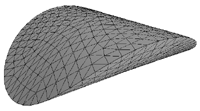Rotation by 90 degrees Ellipse radii: 0.8 and 1.0 [DXF file]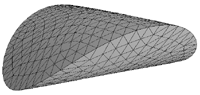Rotation by 22.5 degrees Ellipse radii: 2.0 and 1.0 [DXF file]Rotation by 45 degrees Ellipse radii: 2.0 and 1.0 [DXF file]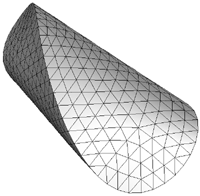Rotation by 90 degrees Ellipse radii: 2.0 and 1.0 [DXF file]Ellipse and circle
 Ellipse radii ratio: 1.5 to 1 [DXF file]Ellipse radii ratio: 2.5 to 1 [DXF file]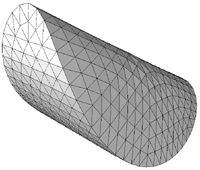Ellipse radii ratio: 4.0 to 1 [DXF file]Miscellaneous
 Circle and curved diamond [DXF file]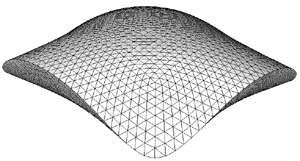Two Triaguloids, 60 degree rotation [DXF file]Circle and rippled outline [DXF file]Two round squares, 30 degree rotation [DXF file]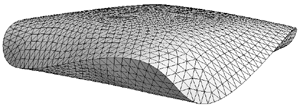Photos by Tony Wills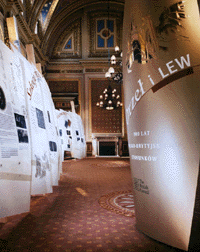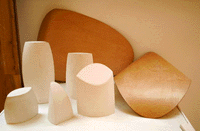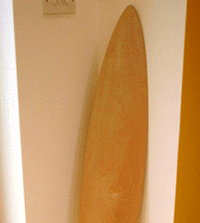Outlines

Experiment with dForms by printing the following outlines and joining them together.